Using Algebraic Operations to Solve Problems

Key Terms

o         Order of operations

o         Substitution

Objectives

o         Review the order of operations in the context of algebra

o         Learn how to manipulate expressions in a way that maintains an equality

o         Understand why substitution can be used in algebra

An important skill in algebra is the ability to perform mathematical manipulations of expressions and equalities. When we deal with simple numbers, such as 2 + 9 = 11, there is seldom a need to make changes to simplify or expand the expressions (2 + 9 and 11 are equivalent expressions). If we deal with an algebraic expression, such as 2 + x = 9, then we must extend our ability to perform arithmetic operations to deal with variables as well as numbers.

Order of Operations

It is helpful to briefly review the order of operations in the context of algebra. When performing any series of operations expressed in mathematical form, perform them in the following order:

1. Any operations in parentheses

2. Exponents

3. Multiplication/division

Within a set of parentheses, there may be a series of operations-in this case, simply start back at the first step for the expression in the parentheses.

Practice Problem: Evaluate the following expression using x = 4: 3x2 - (4x + 1).

Solution: Use the rules for order of operations. First, evaluate the expression in parentheses, using x = 4:

4x + 1 = 4(4) + 1 = 16 + 1 = 17

Next, evaluate the exponent in the first term:

x2 = (4)2 = 16

Let's now take a look at the full expression:

3(16) – 17

Now, evaluate the product of 3 and 16, then subtract 17.

3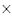16 – 17 = 48 – 17 = 31

The solution is then 31.

Equalities

First, we need to recognize the importance of the "equal sign" (=) in math: if two expressions are equal, then they are indeed equal. Although this fact may seem obvious, it has several important implications for algebra. Let's take the following equality as an example for our discussion: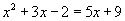Remember, both sides of this equality are equal to one another. As a result, if we perform the same operation, whatever it is, on both sides of the equation, the resulting expressions will still be equal. For instance, if we have two bananas on the left and two bananas on the right, then the numbers of bananas on each side are equal. Thus, if you take one banana away from the left and also take one away from the right, then you still have the same number on both sides. Indeed, if you add x bananas to the left and you add x bananas to the right, you still have the same number on each side, even if you don't know what x is.

So, let's consider our algebraic expression example above. Since both sides are equal, we can do whatever we want to the expressions and still have an equality as long as we perform the same operations, in the same sequence, on both sides. (Note that the sequence is important--the best way to approach this process is to perform one and only one operation on both sides before performing a different operation.) What if we decide to add 2 to both sides?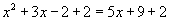The resulting expressions are still equal, because we performed the same operation on both sides. We can even simplify the result as follows (using associativity and commutativity).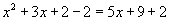Commutativity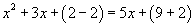Associativity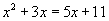These expressions are all still equal. Although the expressions have changed, the equality has not. Note carefully that in this case we have modified the equality so that the constant number value only appears on the right side. Let's do the same for multiples of x by subtracting 3x from both sides of the equality. We will then apply our rules of real numbers.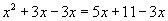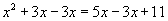Commutativity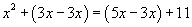Associativity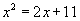So, we can perform operations on both sides of the equal sign regardless of whether they involve just numbers or numbers and variables, and still maintain the equality. Note that the final result above has only three terms total: this is a simpler equality than the one we started with (which had five terms).

We can also perform multiplication and division operations in the same manner. For instance, consider the following equality.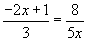Since these expressions are in the form of fractions, they can be difficult to work with. We can simplify them, however, by applying what we know about equalities. Let's first multiply both sides by 5x; the equality will still hold. Again, we'll use our rules for real numbers: associativity, identity, and distributivity.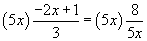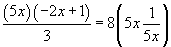Associativity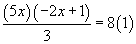Identity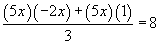Distributivity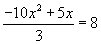So, multiplication and division (note that 5x divided by 5x is just 1) work the same way for algebraic expressions containing numbers as they do for simple numbers. As you become more familiar with these and other algebraic operations, you will become more able to perform them without needing to recognize the specific rules (for instance, associativity) that justify them.

Although it may or may not seem obvious, there is one additional point that we need to consider regarding equalities. Let's say a particular equality contains a variable x. The values of x that satisfy this equality also satisfy other equalities that are derived from this equality using (most) legitimate algebraic operations. (There are some limitations to this statement, but they can typically be addressed case by case.) Let's consider an example: 3 + x = 5. Obviously, the value x = 2 satisfies this equality, since 3 + 2 = 5. What if we perform some algebraic operation, however? Let's add 2 to both sides, then multiply both sides by 5.

3 + x + 2 = 5 + 2

x + 5 = 7

5(x + 5) = 5(7)

5x + 25 = 35

We know that 10 + 25 = 35, so we know that 5x must be equal to 10. Thus, x = 2 once more. We can note from this example that the correct value (or values) for x does not change if the equality is manipulated in a legitimate manner.

Practice Problem: Justify the labeled steps in the following sequence of algebraic manipulations: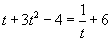Given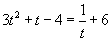A. ?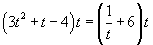Equality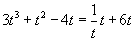B. ?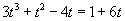C. ?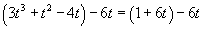Equality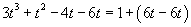D. ?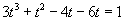E. ?

Solution: To solve this problem, we must recall the basic rules discussed in the previous chapter and apply them to algebra. In the case of A, two terms are reversed, so the justification is commutativity. For B, the factor t is distributed as a factor to each term in parentheses, so this step is justified by distributivity. In C, the product of t and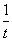is 1, which is the property of (multiplicative) inverses. The step corresponding to D can be justified by associativity, and the step corresponding to E can be justified by (additive) inverses.

Substitution

As noted above, we can make changes to an equality and still maintain the equality. Likewise, we can also substitute one variable or variable expression for another variable or variable expression. For instance, let's say we have the following equality.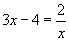Now, let's say that we want to change x into a different form (for whatever reason). Let's say we want x to be called z instead--so, x = z. Then,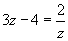is the same as the previous expression. On the other hand, we might say that x = 3y, so that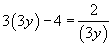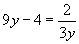Again, this expression maintains the equality. If a particular value of y satisfies this equality, then the corresponding x value (using the relationship x = 3y) also satisfies the preceding expression.

Practice Problem: Substitute p = 3n + 4 into the expression p + 3 –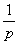.

Solution: The problem statement gives us a new variable expression that we can substitute into the overall expression as follows. Make the substitution for each instance of p. (Note, however, that there is nothing fundamentally wrong with making the substitution for only one instance of p; this leaves us with a new expression containing two variables instead of one. Because the variables are related, however, it is simplest to have the final expression in terms of just one variable.)

p + 3 –= (3n + 4) + 3 –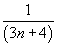We can simplify this result somewhat using our rules of manipulation:

(3n + 4) + 3 –= 3n + (4 + 3) –Associativity

3n + (4 + 3) –= 3n + 7 –This is one potential expression that results from the substitution described in the problem.